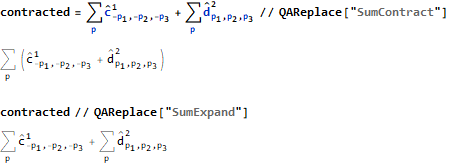## Implementation

The rules for making calculations and simplifications can be divided into different categories:

1. Automatic rules
These are applied automatically after initialization and usually result in a simplification of the expression.
2. "Replacement rules"
There are predefined and freely definable (application specific) substitution rules. A set of such rules is generally summarized under a name. The reason that they are not automatically executed is that the benefits are typically situational. So it may be desirable that sums are summarized or even divided, because one or the other leads to a simplification.3. Assumptions
The Mathematica function Simplify can perform simplifications based on certain assumptions. To make this more manageable, sets of assumptions can be grouped under one name. They can then be referenced under this name.
For example: `exp // QASimplify["Dirac"] `
The expression exp is simplified here on the assumptions of the name "Dirac".
Assumptions are in general application-specific.